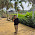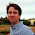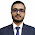Sep 13, 2015

Soldiers in a Line

Source: Alok Goyal's Puzzle Page

Problem:
In a line up of 10 soldiers, what is the least number of soldiers that can be picked in order of either ascending or descending heights? Assume that no two soldiers have the same height. Soldiers can be picked from anywhere in the line, but their order of standing cannot be changed.

1.If one does not pick any soldiers then the answer is 0. In what way are we picking the soldiers ?

2.Assuming we picker can preprocess the heights before selecting, the least number of soldiers that can be picked in either descending or ascending order would be 2.

this scenarios would occur when you pick the first guy as the 5 highest person then try to oscillate between picking the descending and ascending options.

Something like this pattern would occur : /\/\/\/\/

3.4. This is essentially asking to prove the Erdos-Szekeres Theorem.

4.In other words, we want to find the minimum number k such that, for any permutation of 10 elements, we can always either find an increasing subsequence of length k, or a decreasing subsequence of length k.

Given a permutation, we can build a standard Young tableau using the Schensted correspondence:
https://en.wikipedia.org/wiki/Robinson%E2%80%93Schensted_correspondence

This tableau has the property that the length of longest increasing subsequence is the length of the first row (or equivalently, the number of columns), and the length of the longest descreasing subsequence is the height of the first column (or equivalently, the number of lines).

Since a tableau with 10 elements necessarily has either at least 4 lines or at least 4 columns, the number k we are looking for is 4.

More generally, for N soldiers, the numbers is ceil(sqrt(N)).

1.Is there an algorithm for constructing this permutation ?

5.For any general N,answer is the sequence with ceil(sqrt(N)) decreasing subsequences:
(ceil(sqrt(N)),1) , (2*ceil(sqrt(N)),ceil(sqrt(N))+1), ....(i*ceil(sqrt(N)),(i-1)*ceil(sqrt(N))+1) .... (N,(ceil(sqrt(N))-1)*ceil(sqrt(N))+1)
which will essentially give maximum ceil(sqrt(N)) sized increasing/decreasing subsequences.
For example,for N=15: 4 3 2 1 8 7 6 5 12 11 10 9 15 14 13

Fraction Brainteaser

Source: Sent to me by Gaurav Sinha Problem: Siddhant writes a Maths test and correctly answers 5 out of 6 Arithmetic questions and 20...